The Oxford Compendium of Visual Illusions:
Kitaoka, A. (2017). The Fraser-Wilcox illusion and its extension. A. G. Shapiro and D. Todorovi
ć (Eds.), The Oxford Compendium of Visual Illusions, Oxford University Press, pp. 500-511.

since August 9, 2017PDF (scanned copy) --- To buy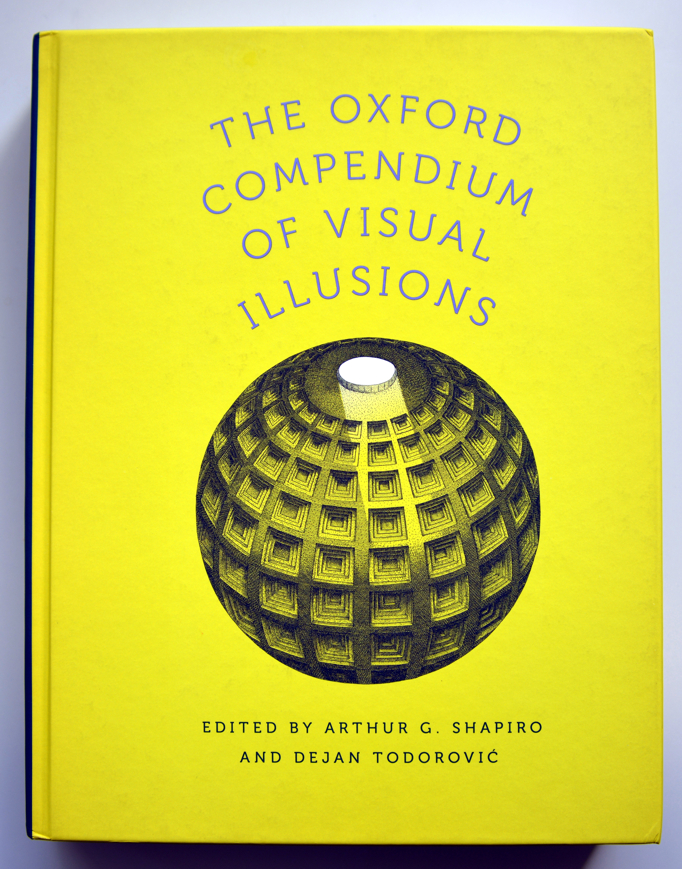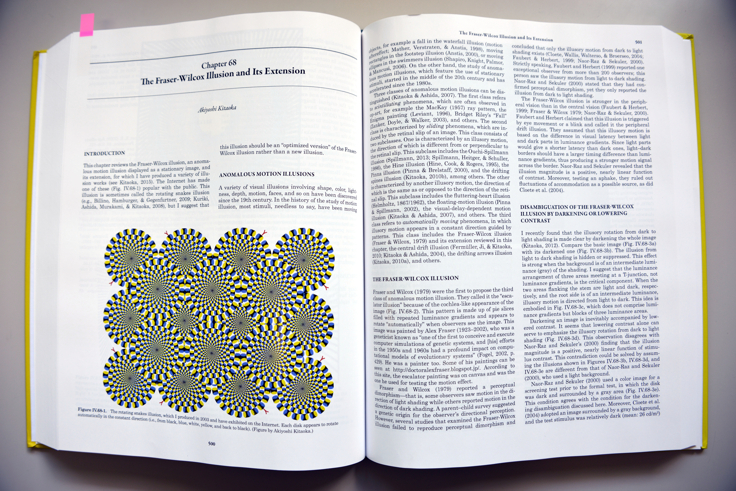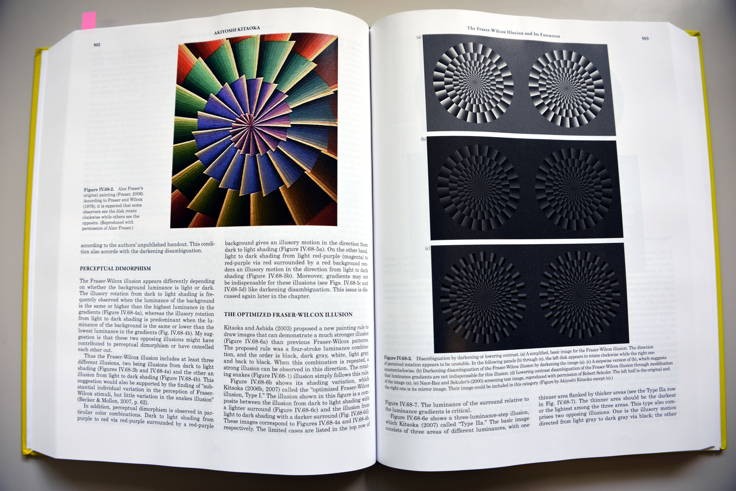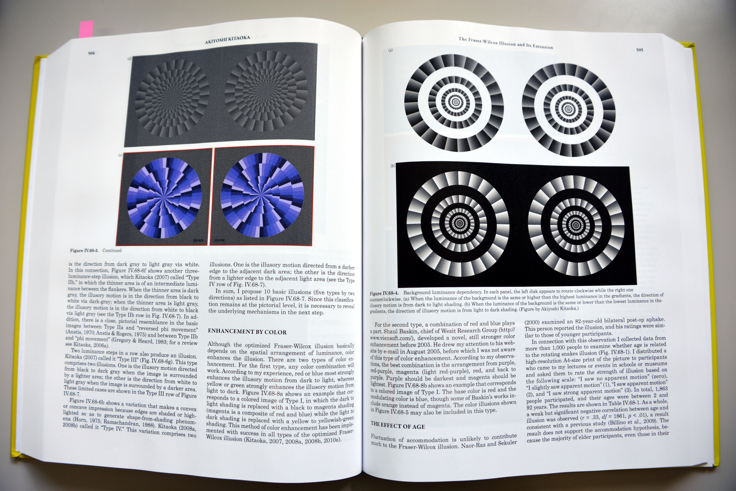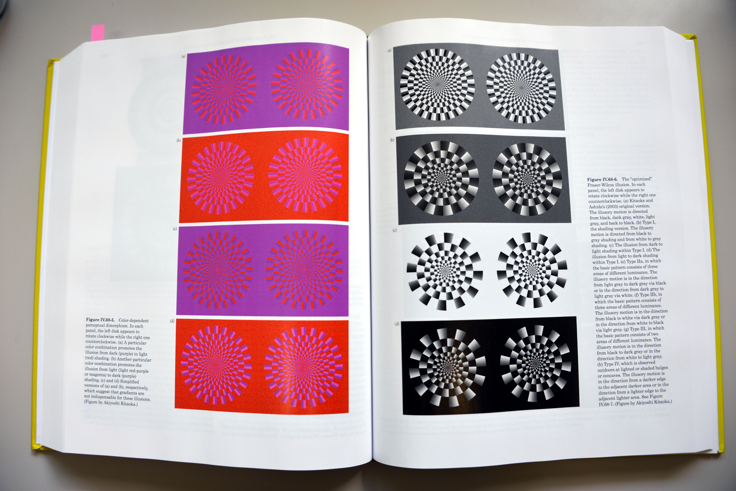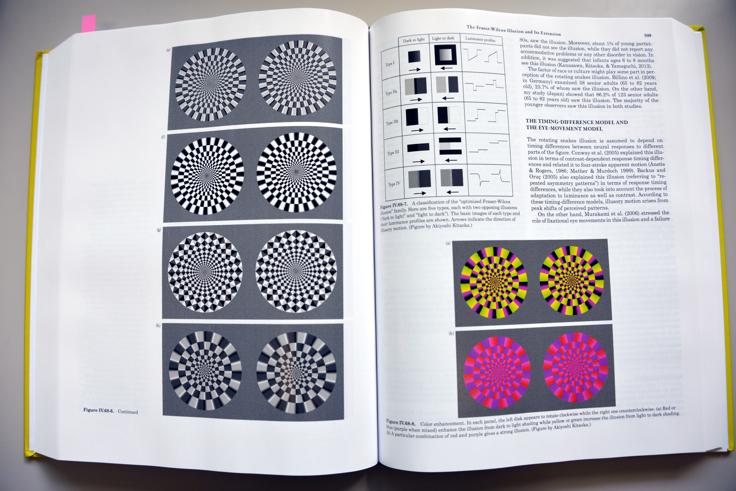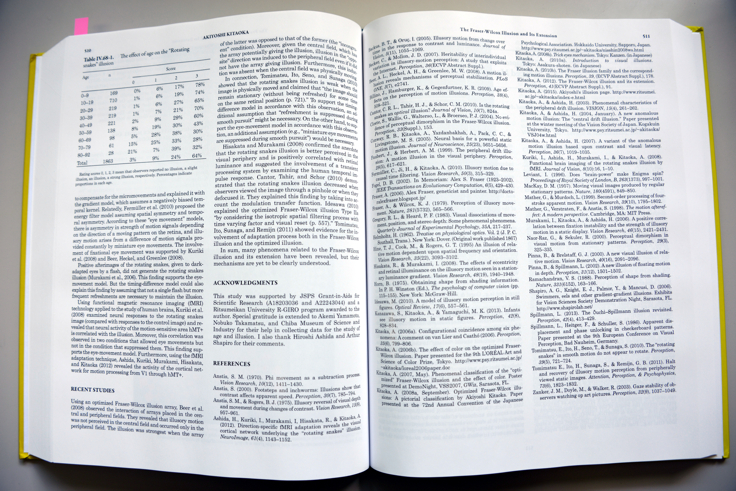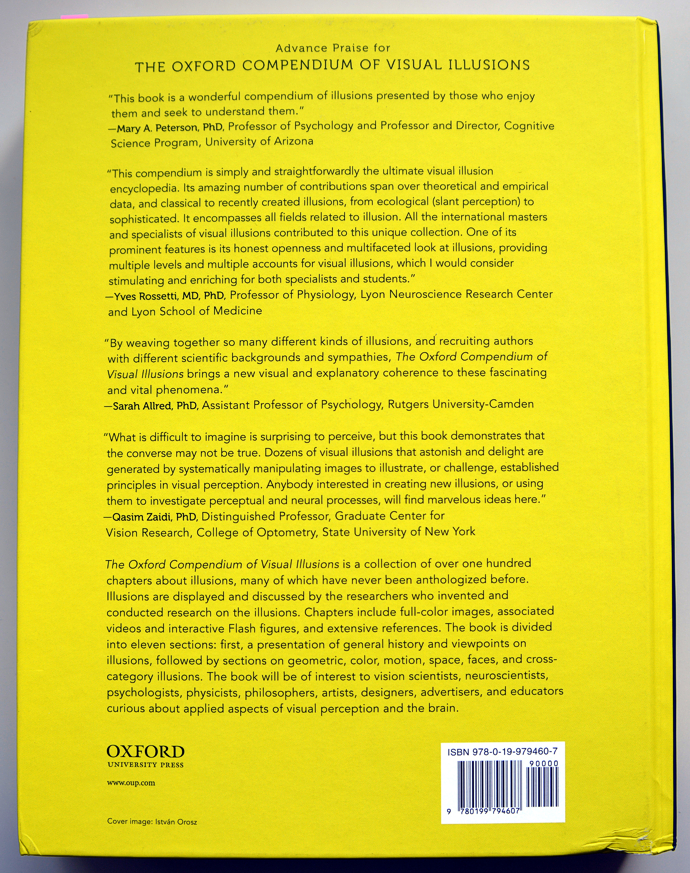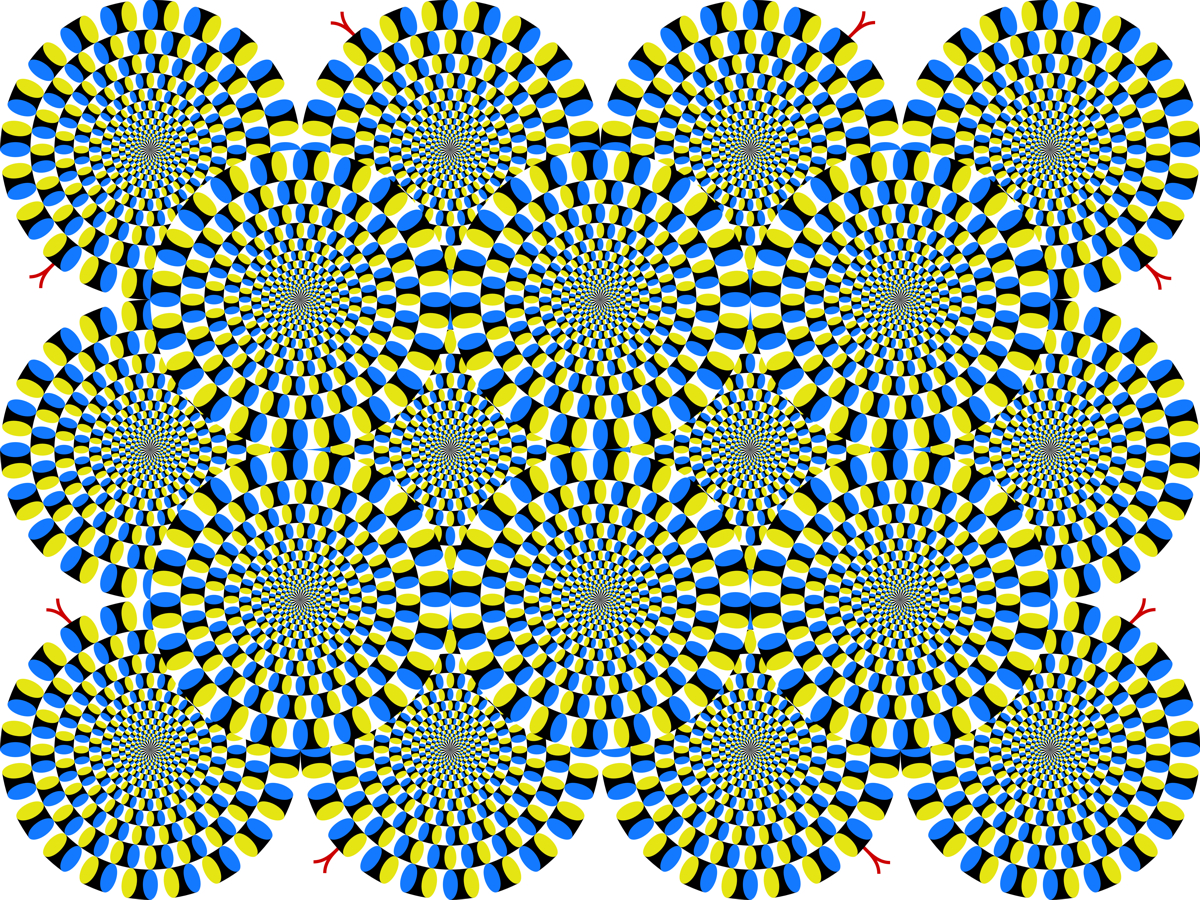Figure 1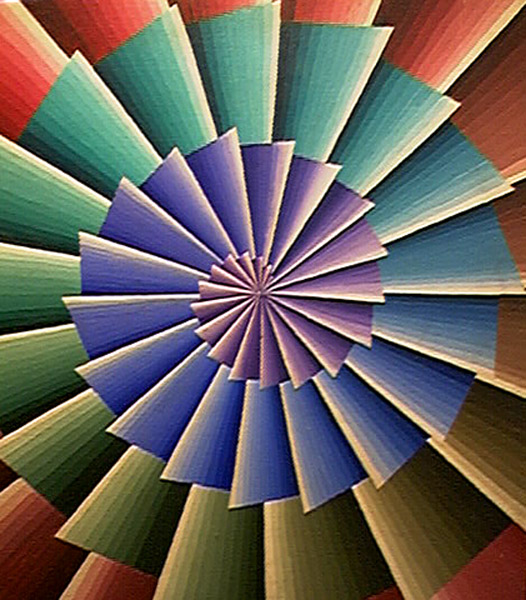Figure 2

Copyright Alan Fraser (the son of Alex Fraser)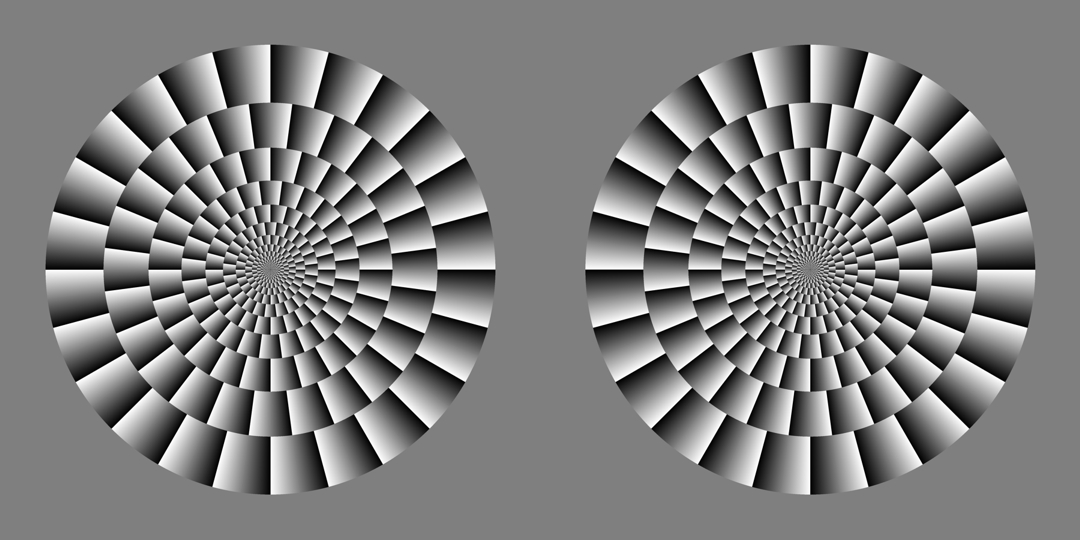Figure 3 (a)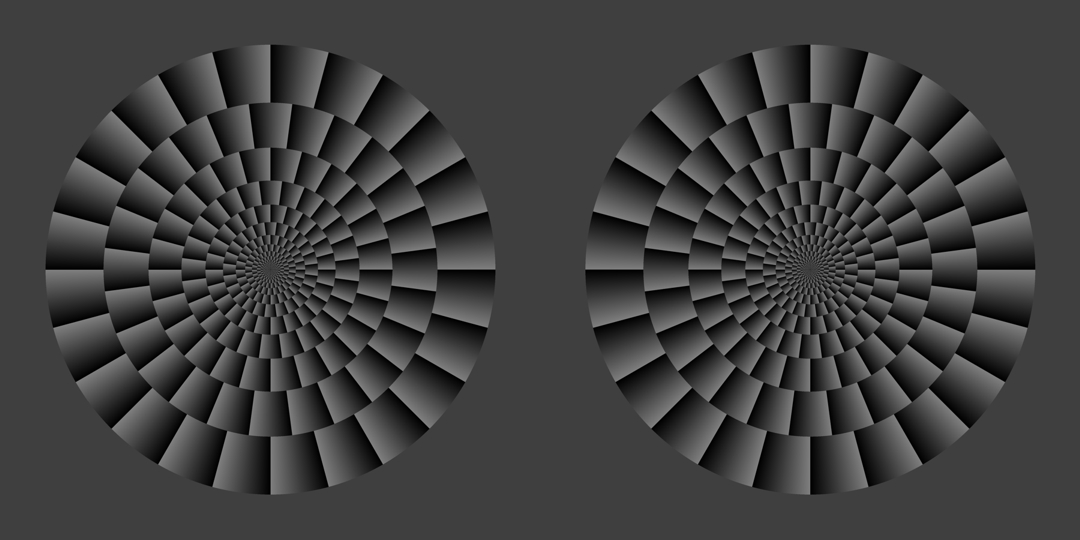Figure 3 (b)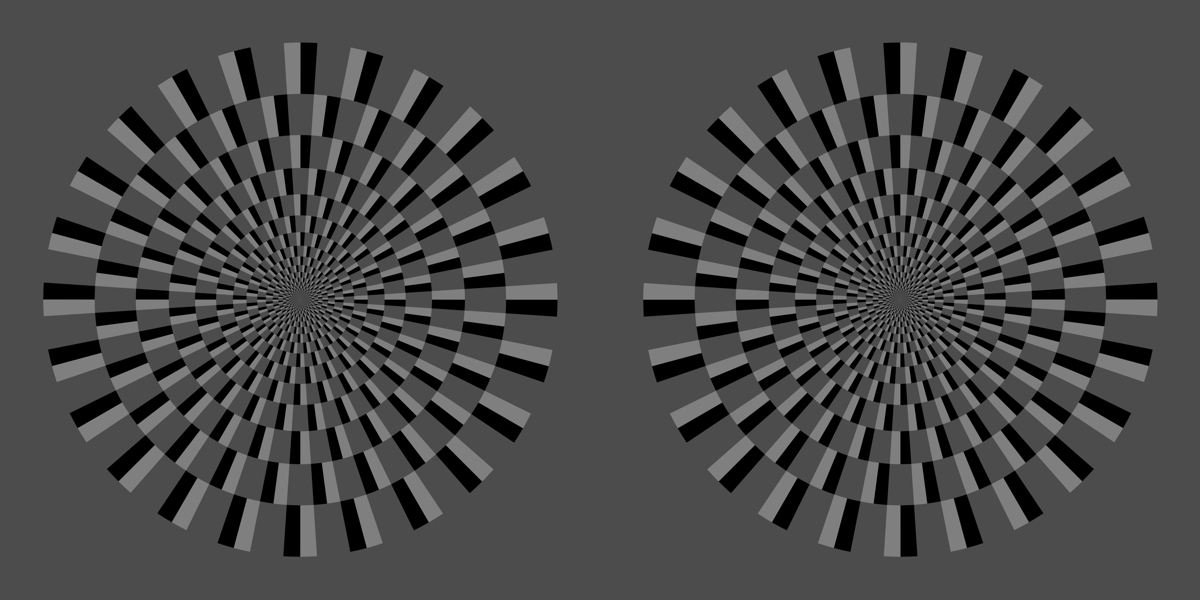Figure 3 (c)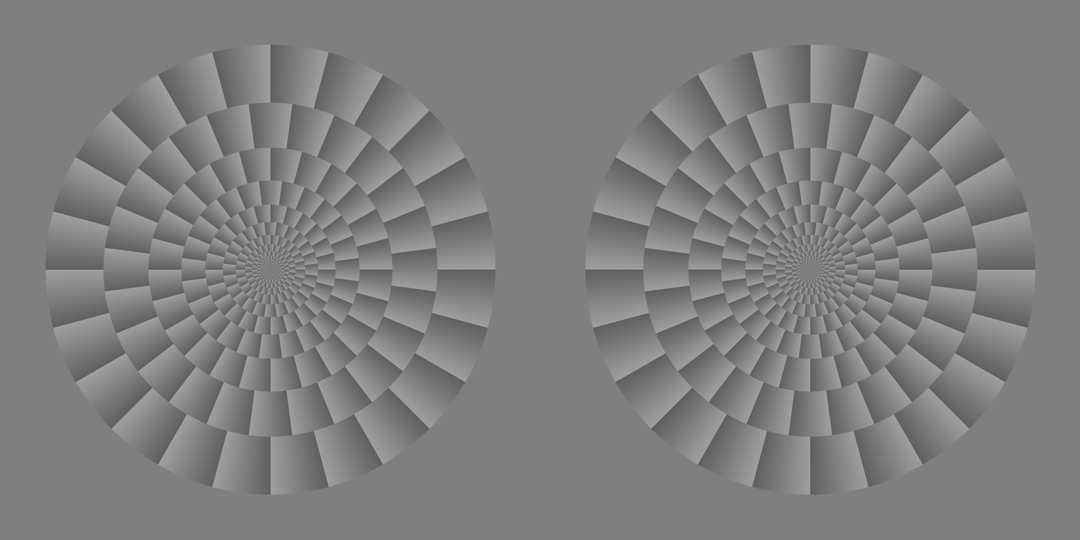Figure 3 (d)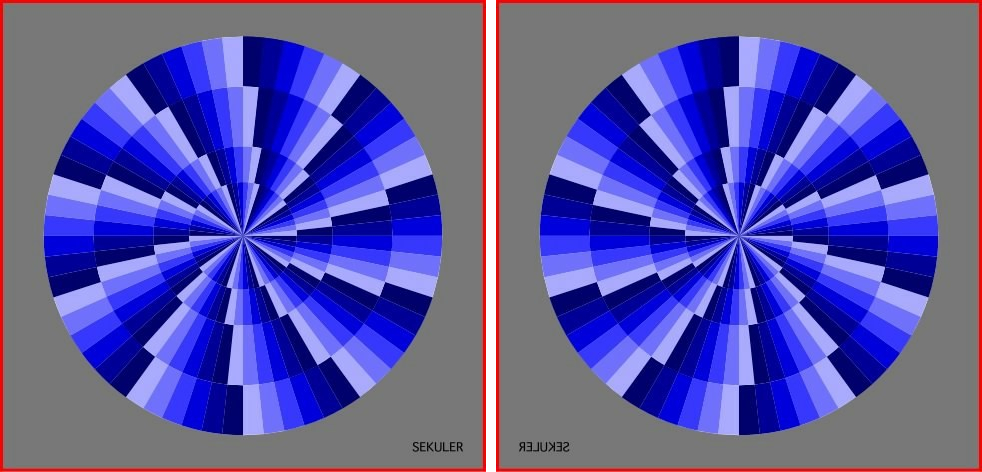Figure 3 (e)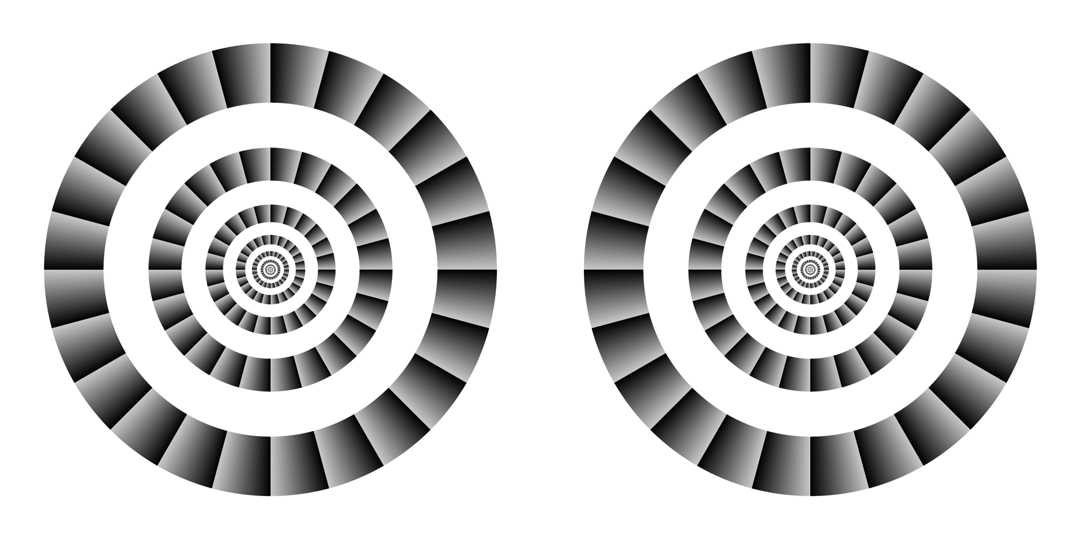Figure 4 (a)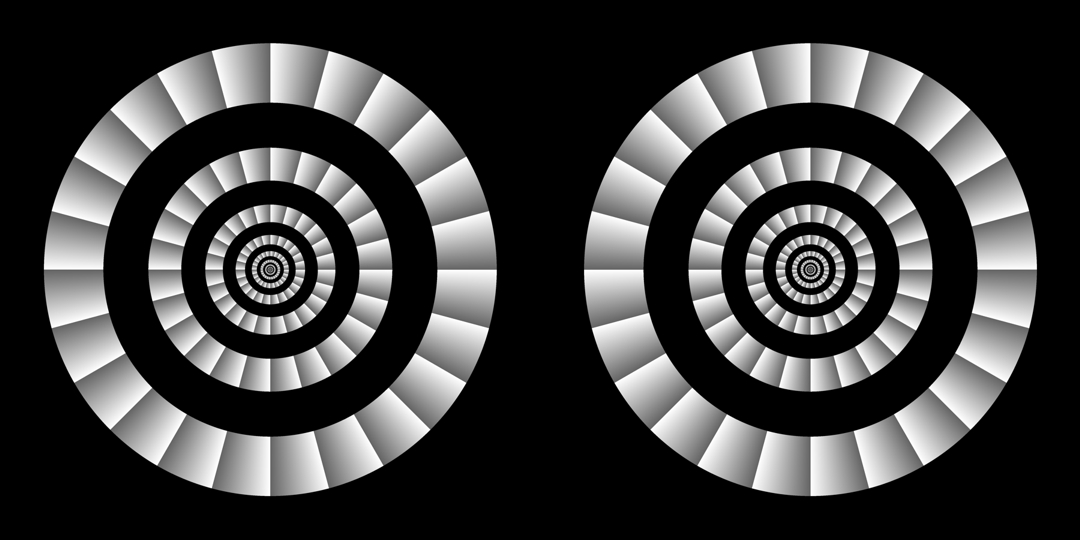Figure 4 (b)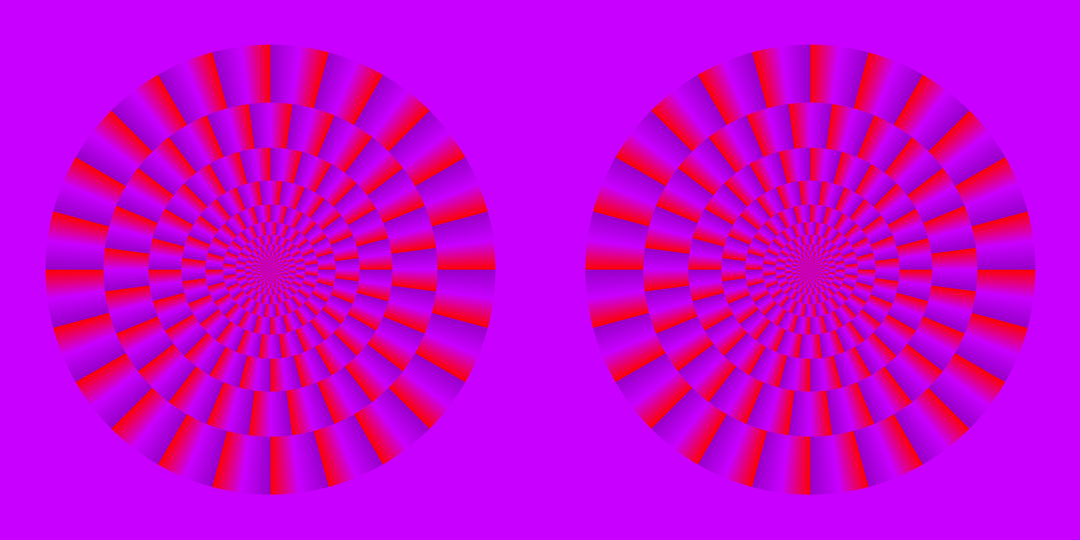Figure 5 (a)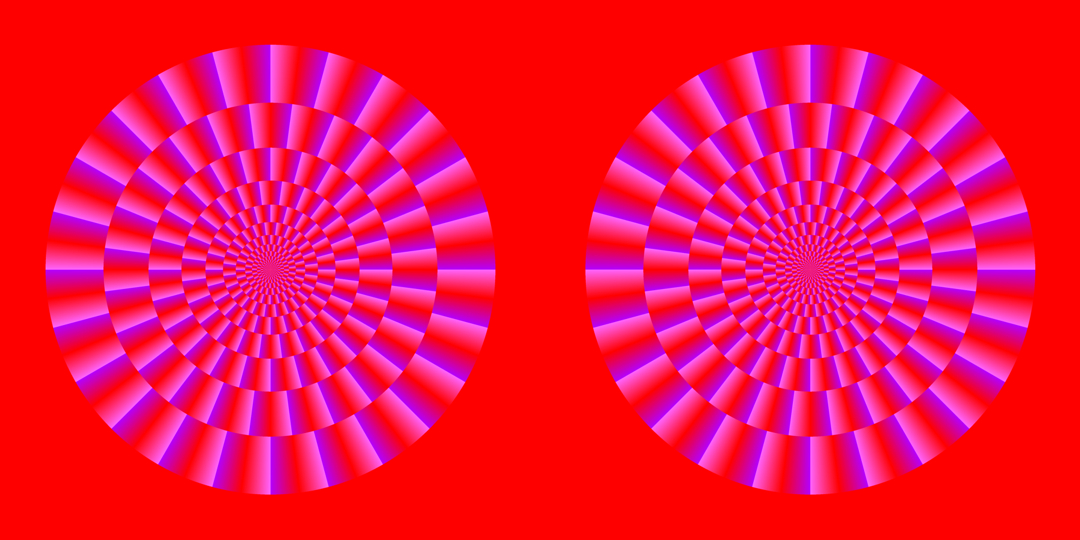Figure 5 (b)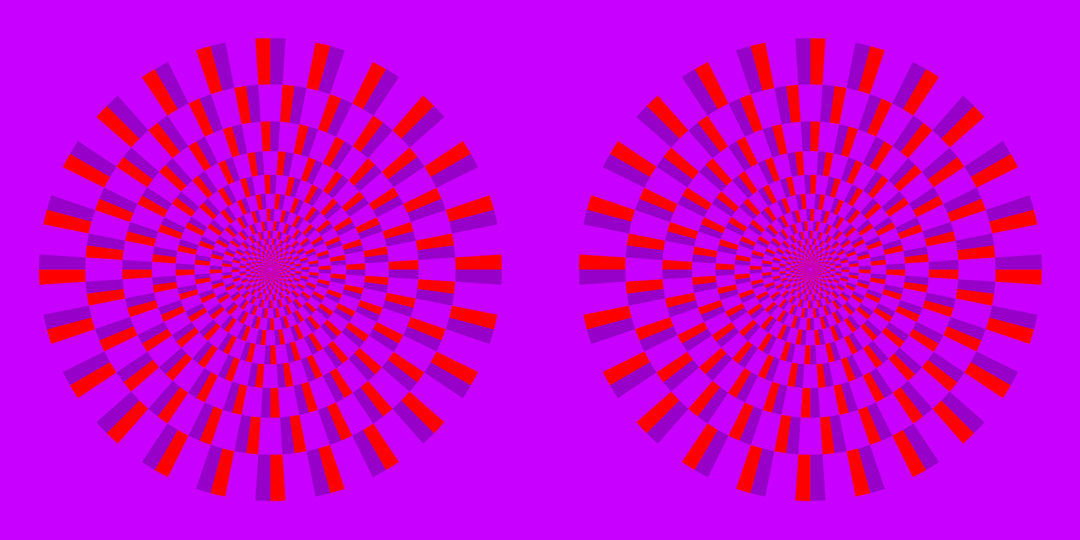Figure 5 (c)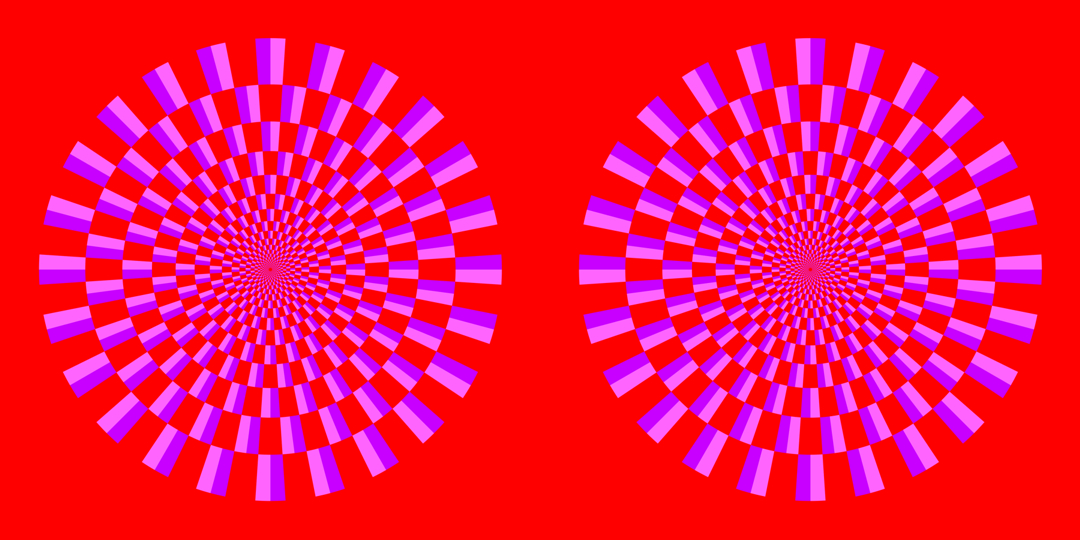Figure 5 (d)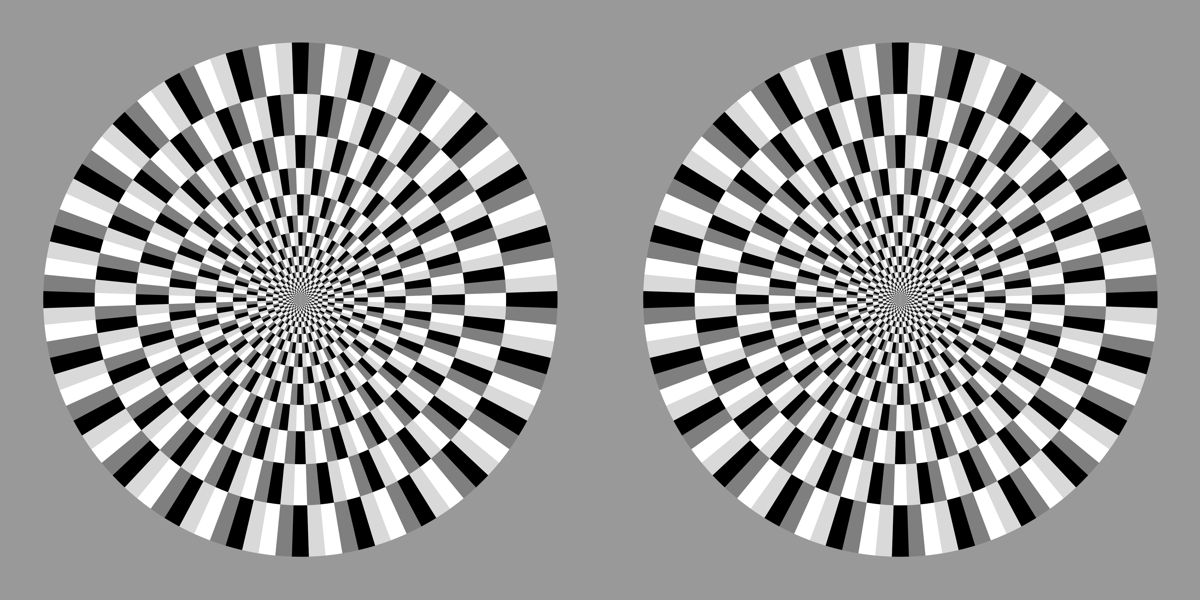Figure 6 (a)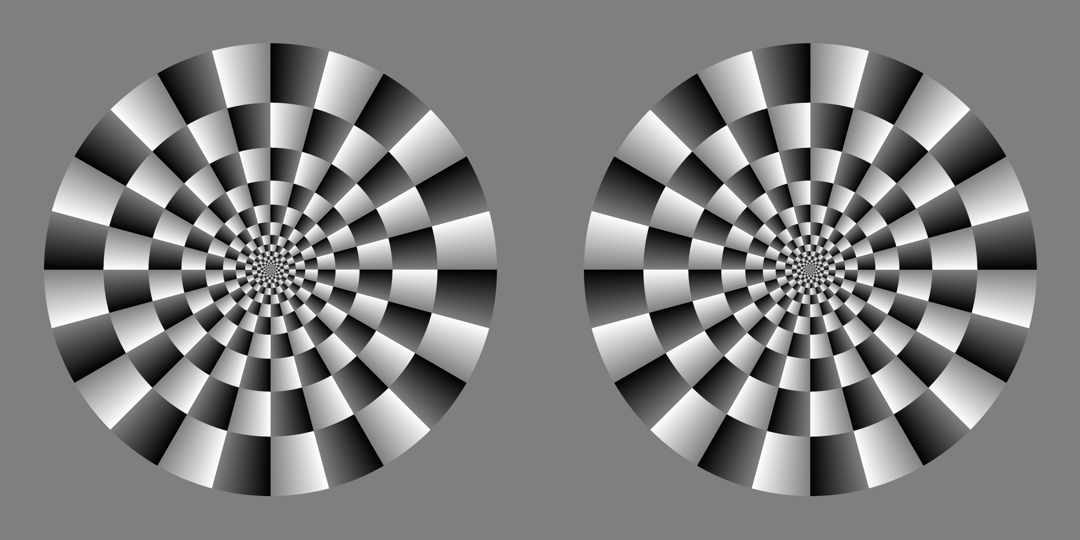Figure 6 (b)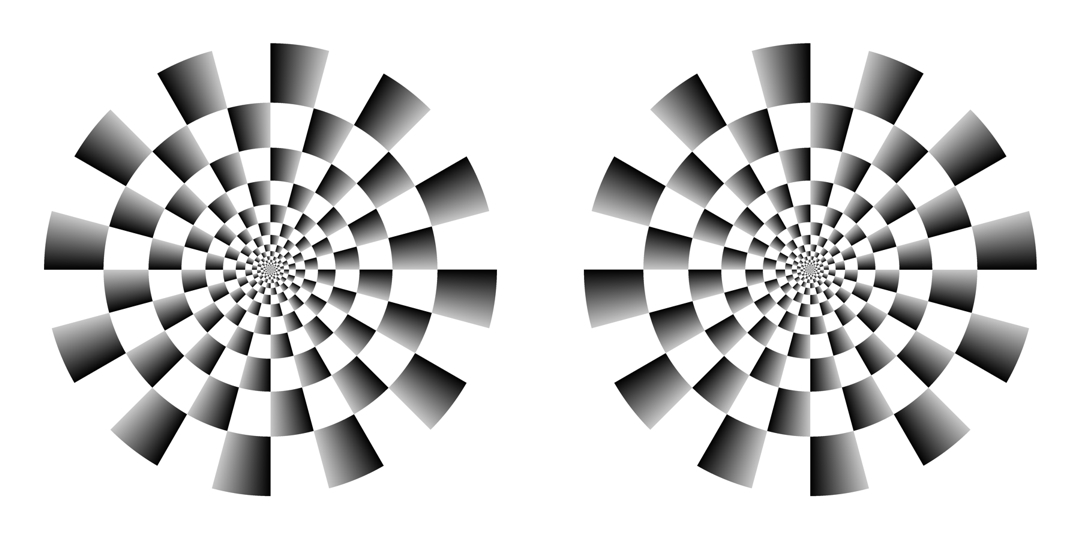Figure 6 (c)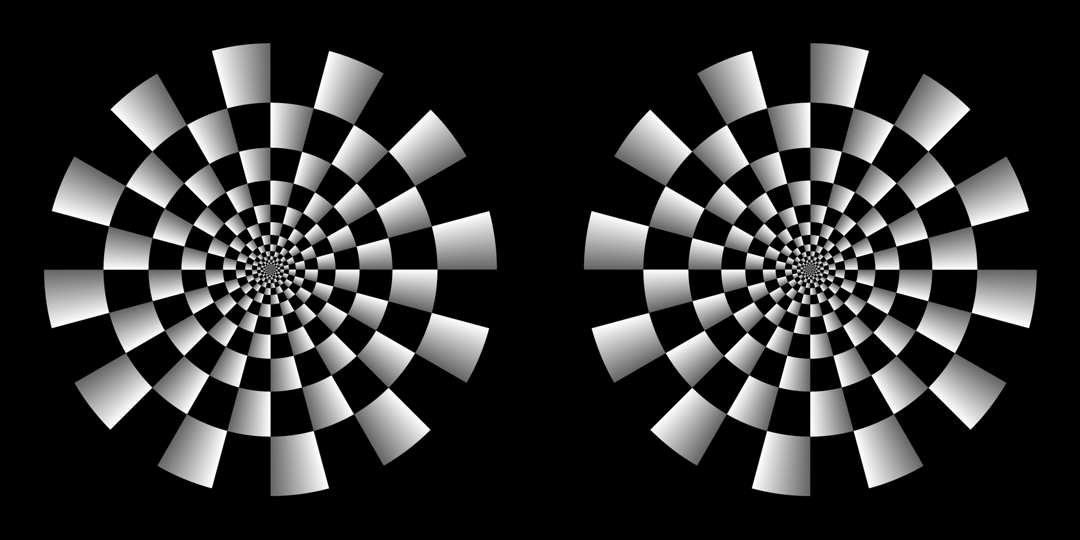Figure 6 (d)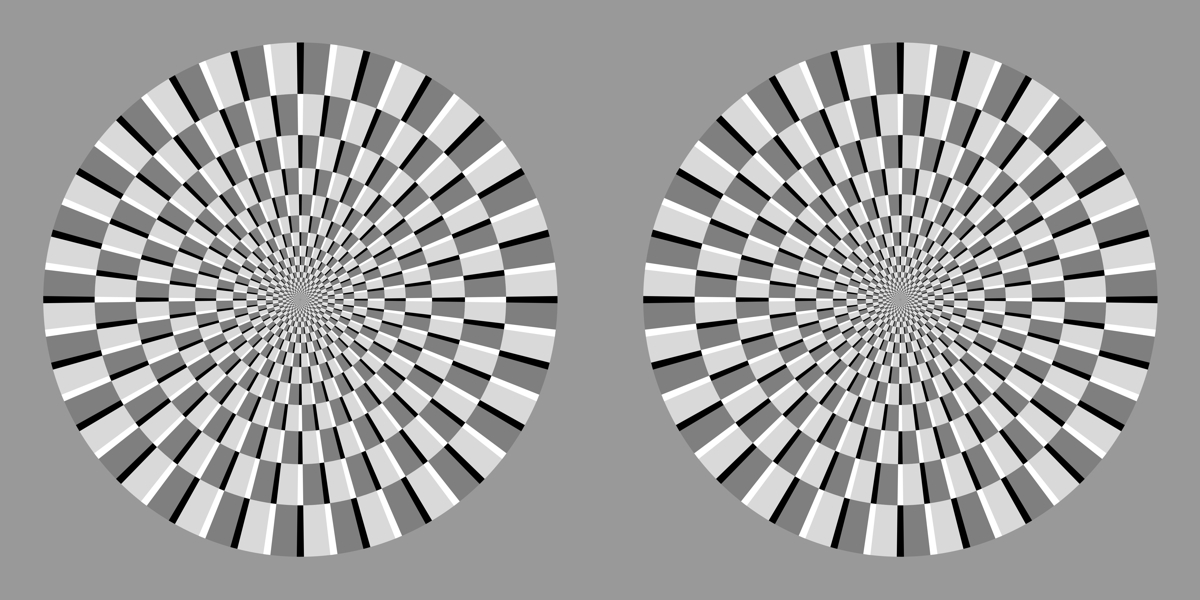Figure 6 (e)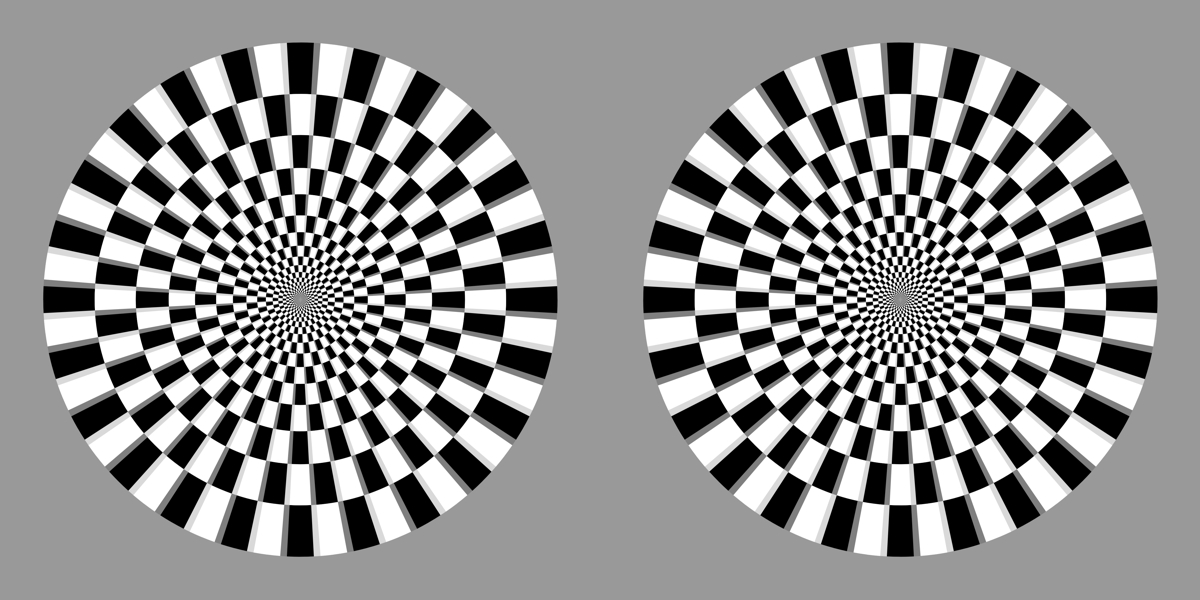Figure 6 (f)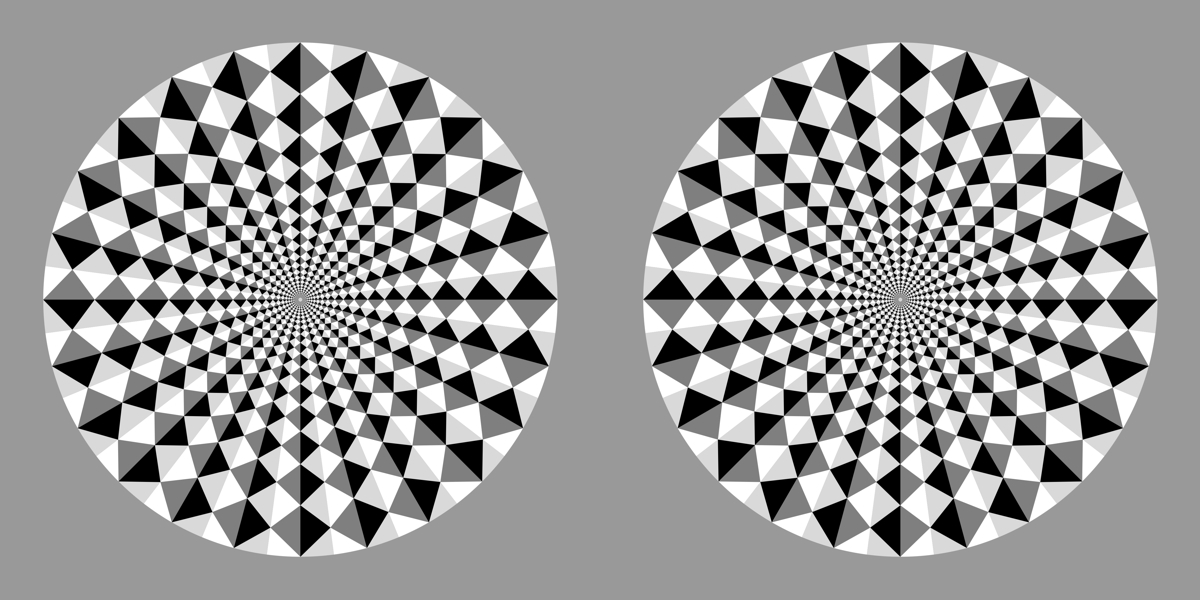Figure 6 (g)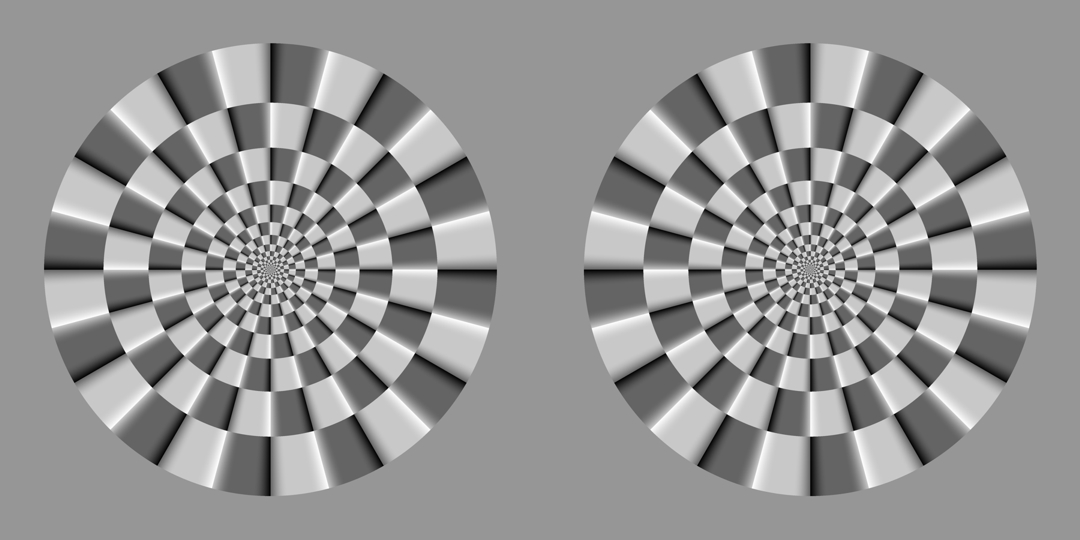Figure 6 (h)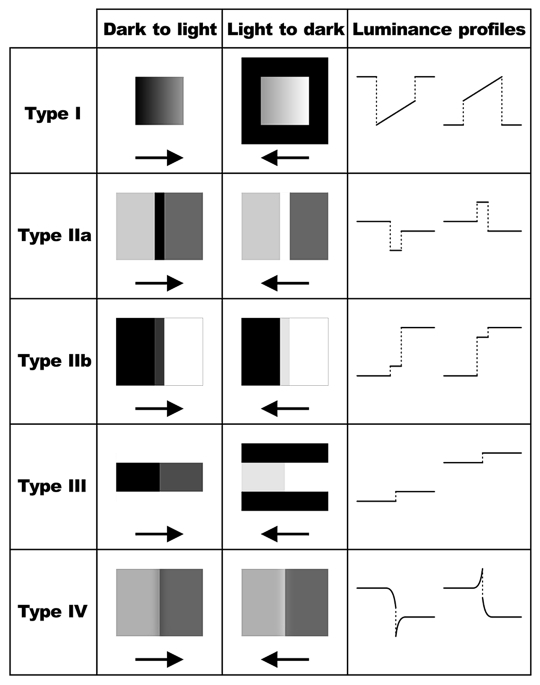Figure 7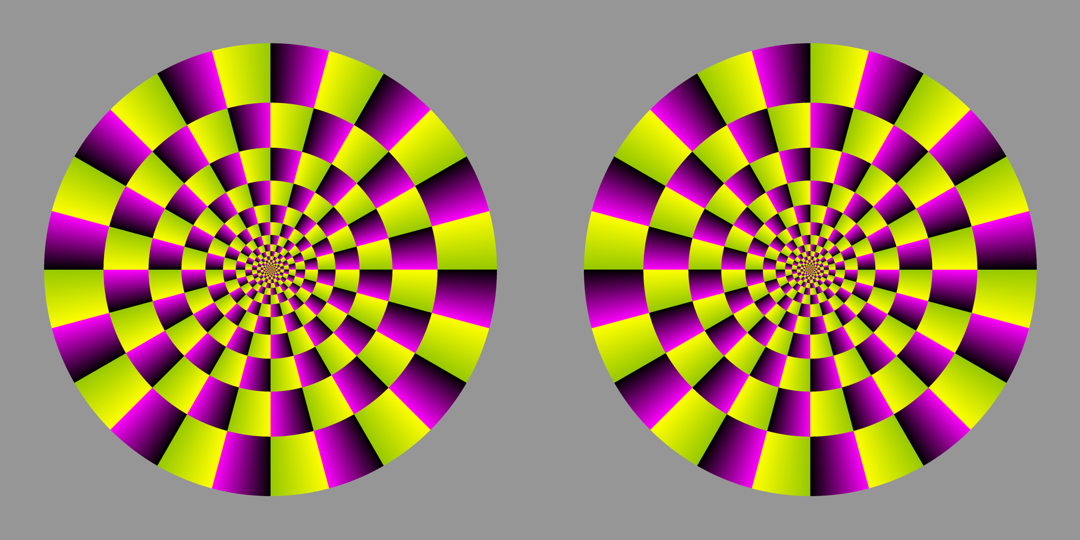Figure 8 (a)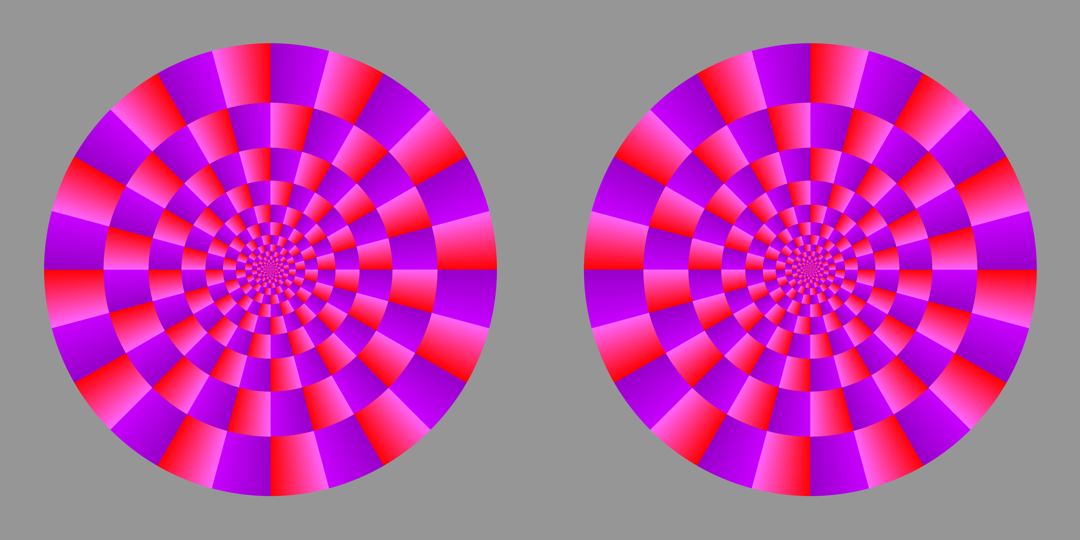Figure 8 (b)

Akiyoshi's illusion pages